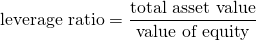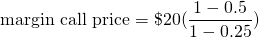# Margin call price

The margin call price is the stock price at which we can expect a margin call when we employ leverage. It is important to keep this price in mind when holding leveraged positions.

On this page, we explain the necessary concepts to be able to calculate the margin call price. In particular, we discuss the margin call definition, compare initial margin vs maintenance margin, provide the margin call price formula and discuss an example. Finally, an Excel spreadsheet that implements a simple margin call calculator. The spreadsheet can be found at the end of this page.

## Margin call definition

A margin call occurs when an investors buys stocks on margin. This means that, rather than financing the whole stock positions using equity, the investor borrows money from the broker. This money is then used to buy more of the stock than would otherwise be possible. The amount of leverage is then the total value of the position divided by the equity positionTo ensure that the investor will be able to repay the loan received by the broker, the investor must maintain a minimum equity percentage. The initial amount when the position is opened is called the initial margin. Once the position open, the amount required is the maintenance margin requirement. The margin call price refers to the price at which the investor will be requested to bring the equity back to the maintenance margin percentage.

## Margin call example

Let us consider a margin call example. Suppose an investors buys a stock for $20 per share with an initial margin of 50% and a maintenance margin of 25%. What is the margin call price. Using the above formula, we getor$13.33. Clearly, once we have the initial margin and maintenance margin percentages, it is very easy to determine the price at which we can expect to get a margin call from the broker. Note that, in the case of a short sale, the investor also posts margin equal to a percentage of the value of the shares he or she sold short. This margin is to protect the broker from losses when the stock price goes up.

### Margin call price calculator

Want to have an implementation in Excel? Download the Excel file: Margin Call Price